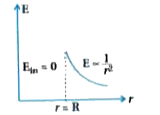Doubtnut App - Get 50 Lakh+ video solutions better than GoogleInstall Now

# A hollow metal sphere of radius R is uniformly charged. The electric field due to the sphere at a distance r from the centreSolution in Gujrati

A

increases as r increases for r lt R and for r gt R

B

zero as r increases for r lt R, decreases as r increases for r gt R.

C

zero as r increases for r lt R, increases as r increases for r gt R.

D

decreases as r increases for r lt R and for r gt R.

Text Solution
Verified by Experts

## Electric field inside hollow sphere is zero and outside is =kqr2